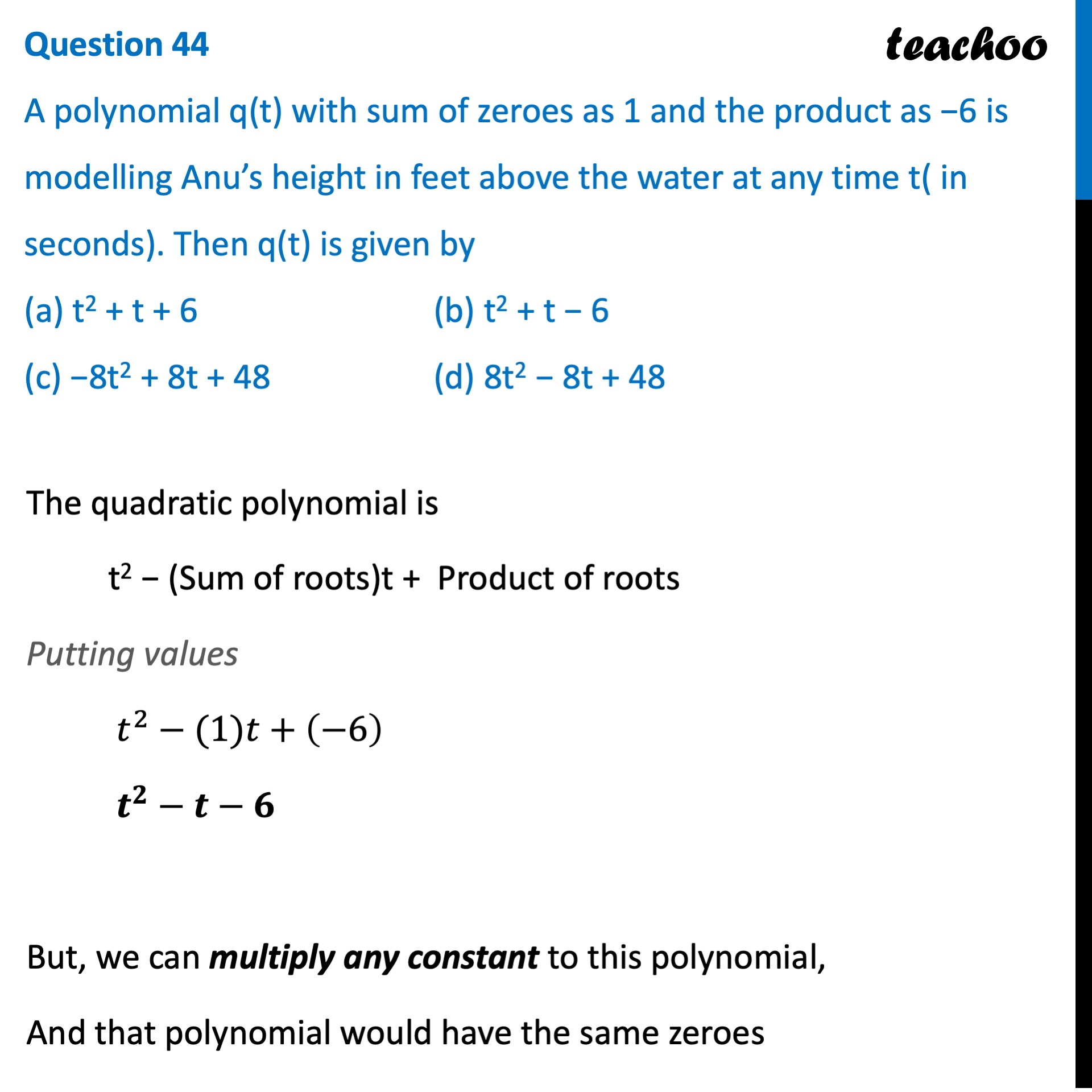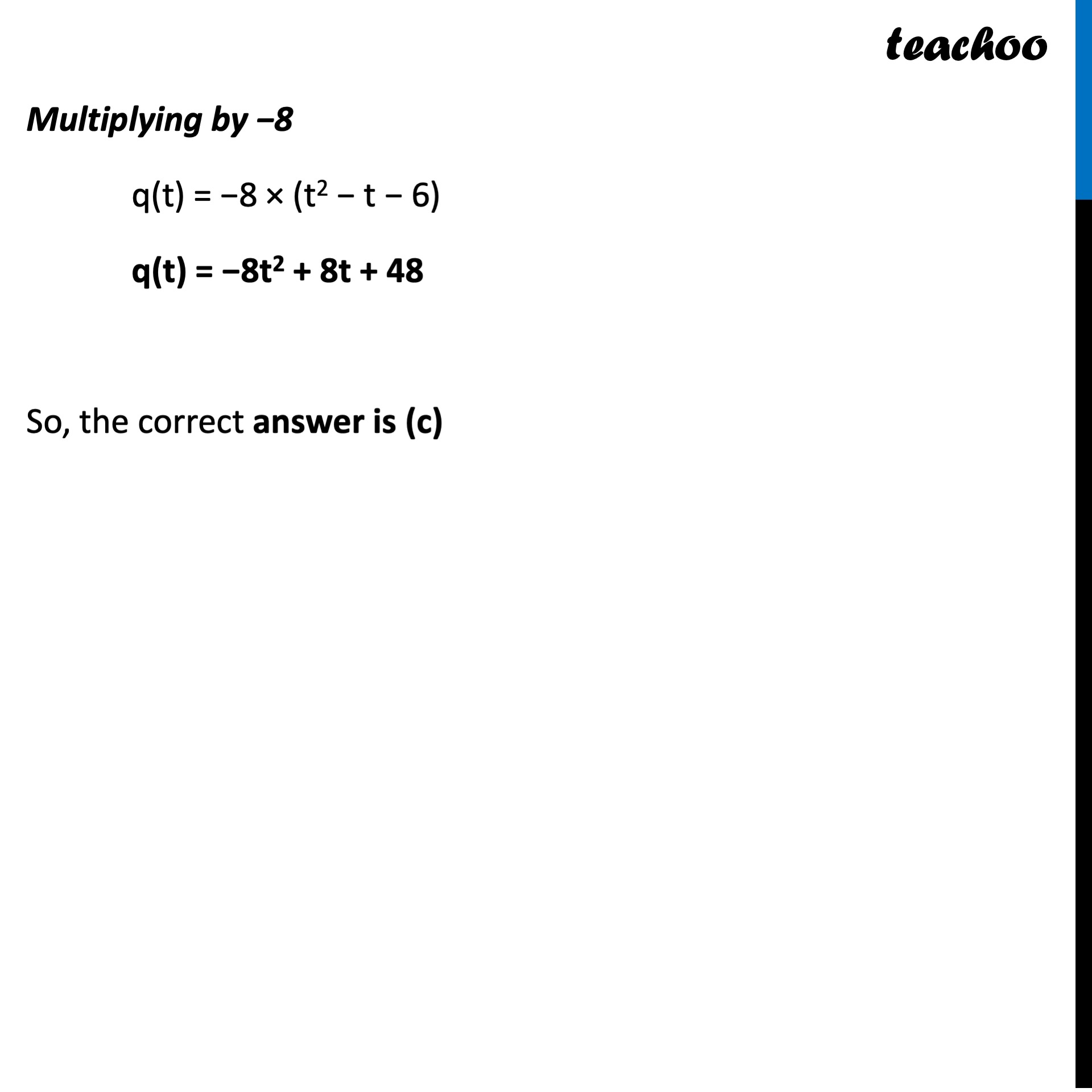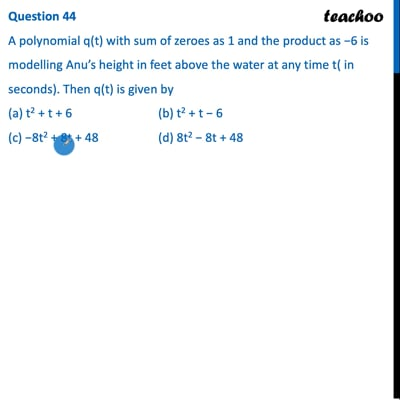CBSE Class 10 Sample Paper for 2022 Boards - Maths Standard [MCQ]

Class 10
Solutions of Sample Papers for Class 10 Boards

## (c) −8t 2 + 8t + 48   (d) 8t 2 − 8t + 48This video is only available for Teachoo black users

Introducing your new favourite teacher - Teachoo Black, at only ₹83 per month

### Transcript

Question 44 A polynomial q(t) with sum of zeroes as 1 and the product as −6 is modelling Anu’s height in feet above the water at any time t( in seconds). Then q(t) is given by (a) t2 + t + 6 (b) t2 + t − 6 (c) −8t2 + 8t + 48 (d) 8t2 − 8t + 48 The quadratic polynomial is t2 − (Sum of roots)t + Product of roots Putting values 𝑡^2−(1)𝑡+(−6) 𝒕^𝟐−𝒕−𝟔 But, we can multiply any constant to this polynomial, And that polynomial would have the same zeroes Multiplying by −8 q(t) = −8 × (t2 − t − 6) q(t) = −8t2 + 8t + 48 So, the correct answer is (c)